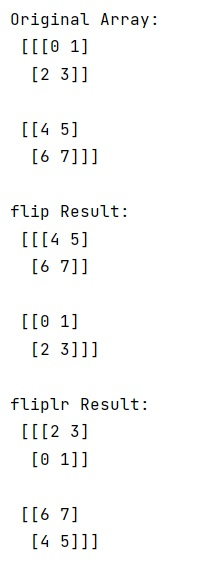# Difference between flip() and fliplr() functions in NumPy

Learn about the difference between flip() and fliplr() functions in Python NumPy.
Submitted by Pranit Sharma, on February 28, 2023

NumPy is an abbreviated form of Numerical Python. It is used for different types of scientific operations in python. Numpy is a vast library in python which is used for almost every kind of scientific or mathematical operation. It is itself an array which is a collection of various methods and functions for processing the arrays.

## numpy.flip() Vs numpy.fliplr() functions

In NumPy, the numpy.flip() function is used to reverse the order of elements in an array along the given axis. Here, the shape of the array is preserved, but the elements are reordered. It takes a specified axis or axes as a parameter along which to flip over the order of elements.

On the other hand, numpy.fliplr() function is used to reverse the order of elements along axis 1 (left/right). Here, it is fixed that the elements will be reversed along axis 1.For a 2-D array, this flips the entries in each row in the left/right direction. Columns are preserved but appear in a different order than before.

Let us understand with the help of an example,

## Python code to demonstrate the difference between flip() and fliplr() functions in NumPy

```# Import numpy
import numpy as np

# Creating a numpy array
arr = np.arange(8).reshape((2,2,2))

# Display original array
print("Original Array:\n",arr,"\n")

# using flip
res = np.flip(arr, 0)

# Display result
print("flip Result:\n",res,"\n")

# using fliplr
res = np.fliplr(arr)

# Display result
print("fliplr Result:\n",res)
```

Output:Languages: » C » C++ » C++ STL » Java » Data Structure » C#.Net » Android » Kotlin » SQL
Web Technologies: » PHP » Python » JavaScript » CSS » Ajax » Node.js » Web programming/HTML
Solved programs: » C » C++ » DS » Java » C#
Aptitude que. & ans.: » C » C++ » Java » DBMS
Interview que. & ans.: » C » Embedded C » Java » SEO » HR
CS Subjects: » CS Basics » O.S. » Networks » DBMS » Embedded Systems » Cloud Computing
» Machine learning » CS Organizations » Linux » DOS
More: » Articles » Puzzles » News/Updates